## 前言

• 顾名思义，快速幂就是快速算底数的n次幂。

• 其时间复杂度为 O(log₂n)， 与朴素的O(n)相比效率有了极大的提高。

• 快速幂属于数论的范畴，本是ACM经典算法，但现在各厂对算法的要求越来越高，并且快速幂适用场景也比较低多并且相比朴素方法有了非常大的提高。所以掌握快速幂算法已经是一名更合格的工程师必备要求！

## 快速幂介绍

### 初探

``````int va=1;
for(int i=0;i<10;i++)
{
va*=2;
}
System.out.println(va%10000);

``````long va=1;
for(int i=0;i<50;i++)
{
va*=2;
}
System.out.println(va);
System.out.println(va%10000);

``````(a + b) % p = (a % p + b % p) % p  （1）
(a - b) % p = (a % p - b % p ) % p （2）
(a * b) % p = (a % p * b % p) % p  （3）
a ^ b % p = ((a % p)^b) % p        （4）

``````(a * b) % p = (a % p * b % p) % p   (3)
(2*2*2···*2) %1e10=[2*(2*2···*2)]%1e5=(2%1e5)*(2*2···*2%le5)%1e5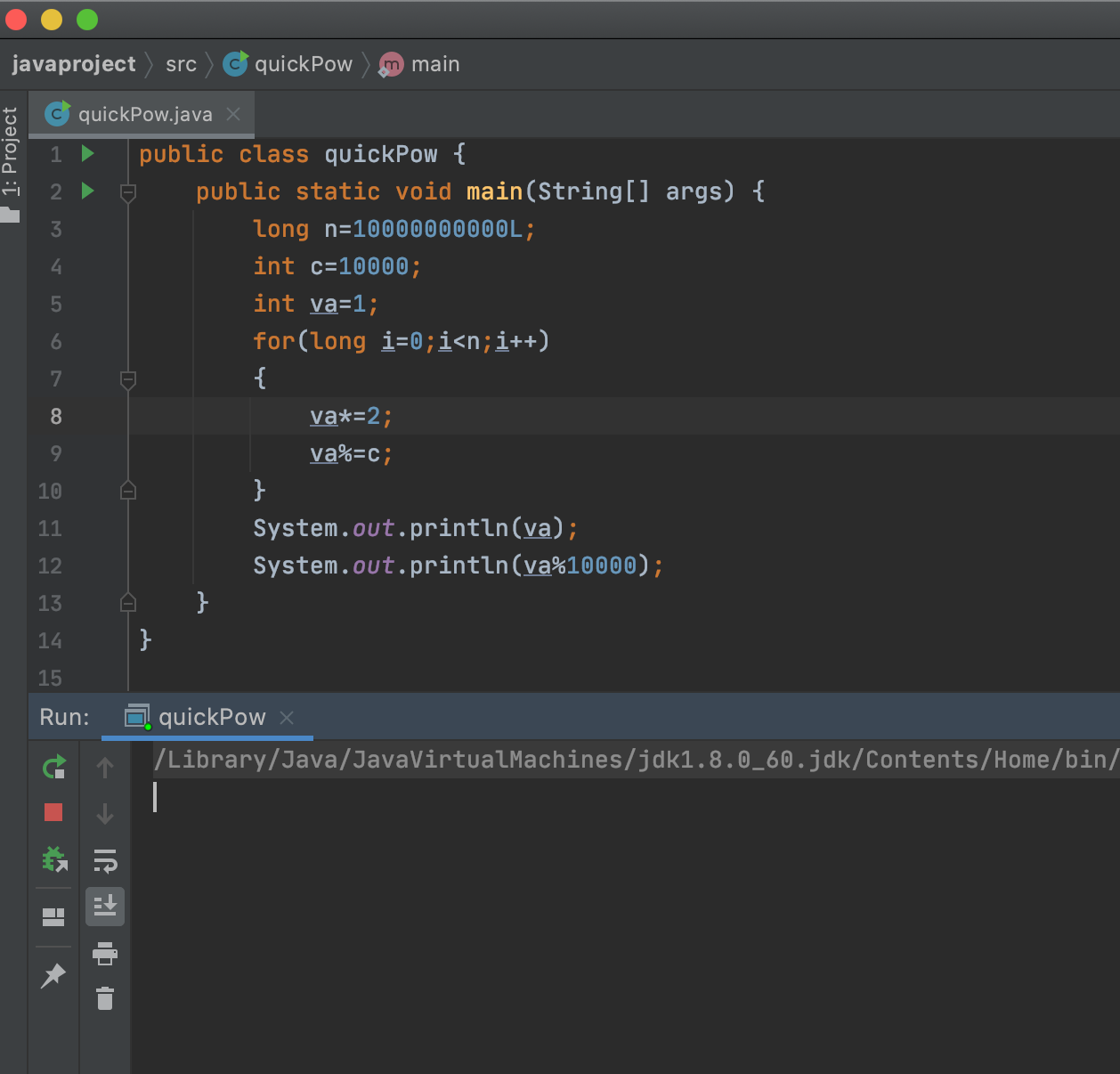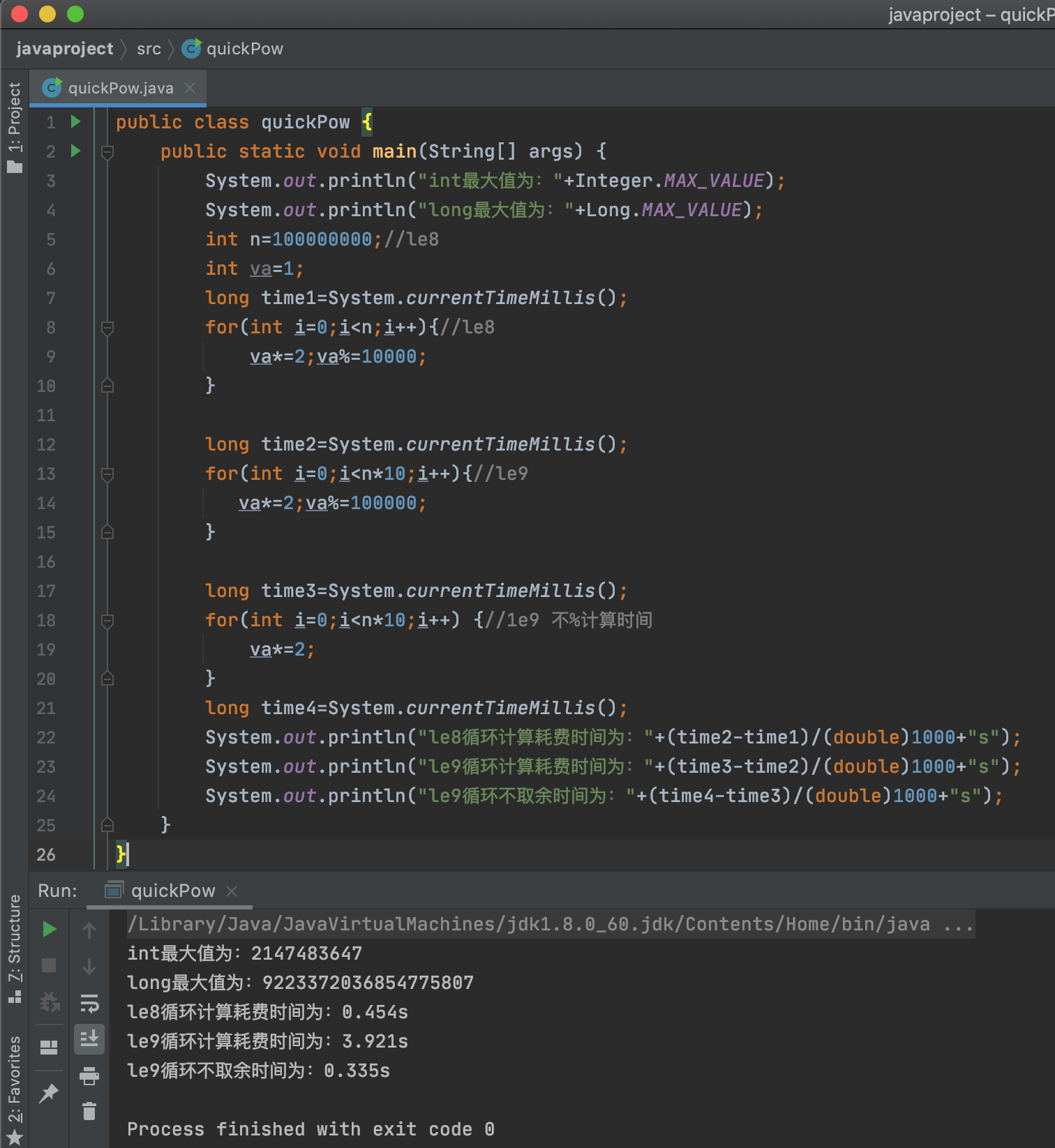### 快速幂探索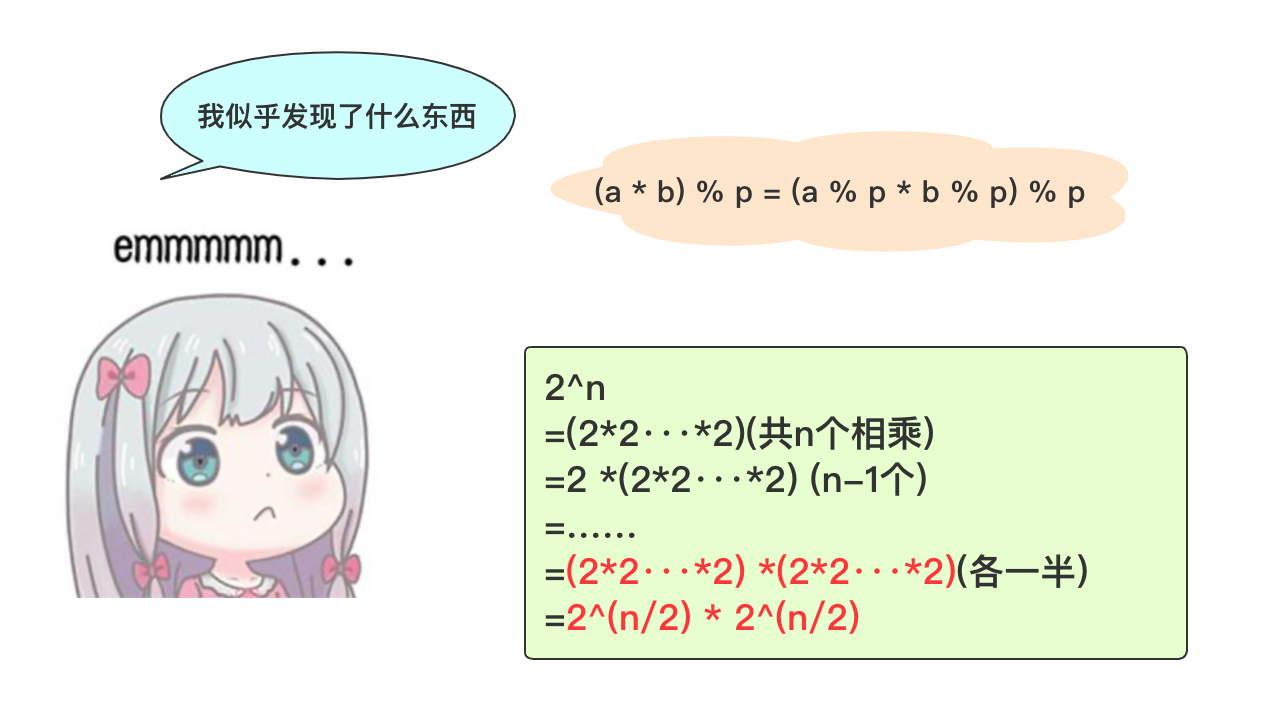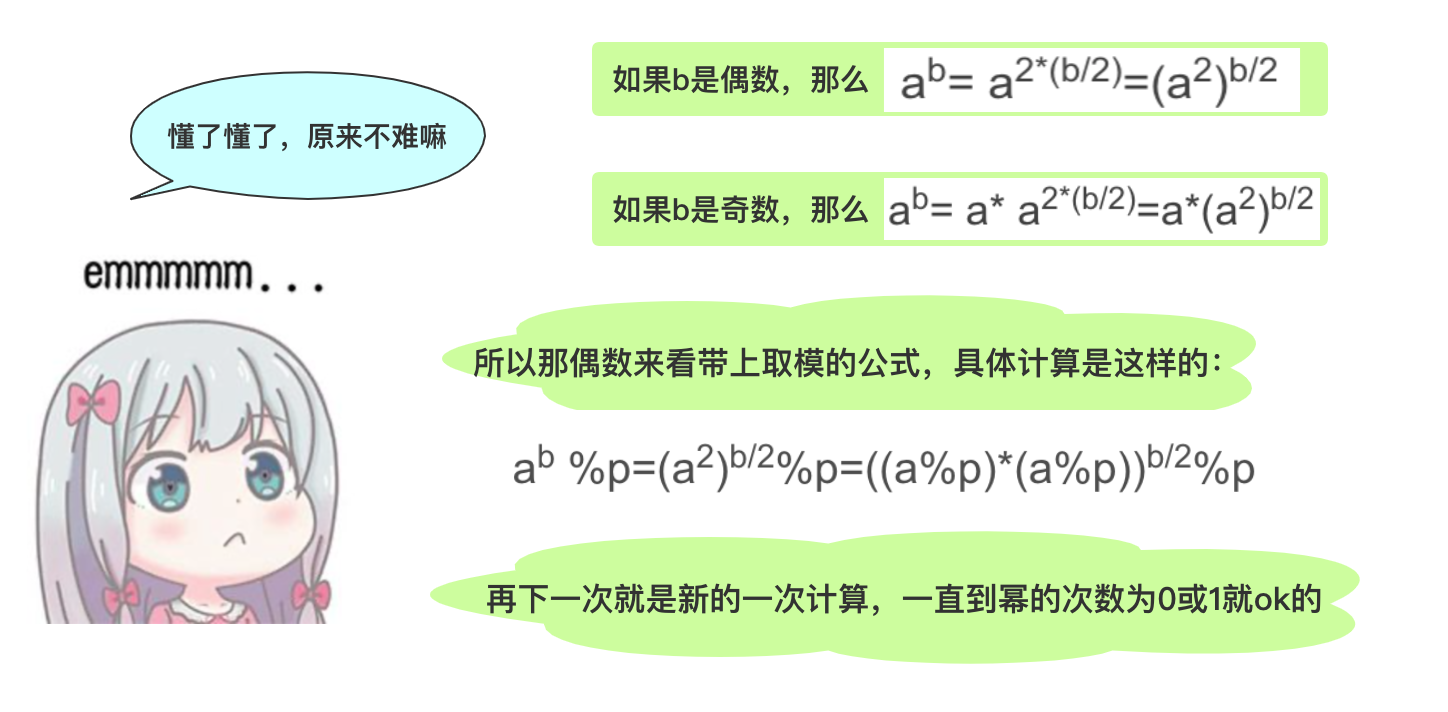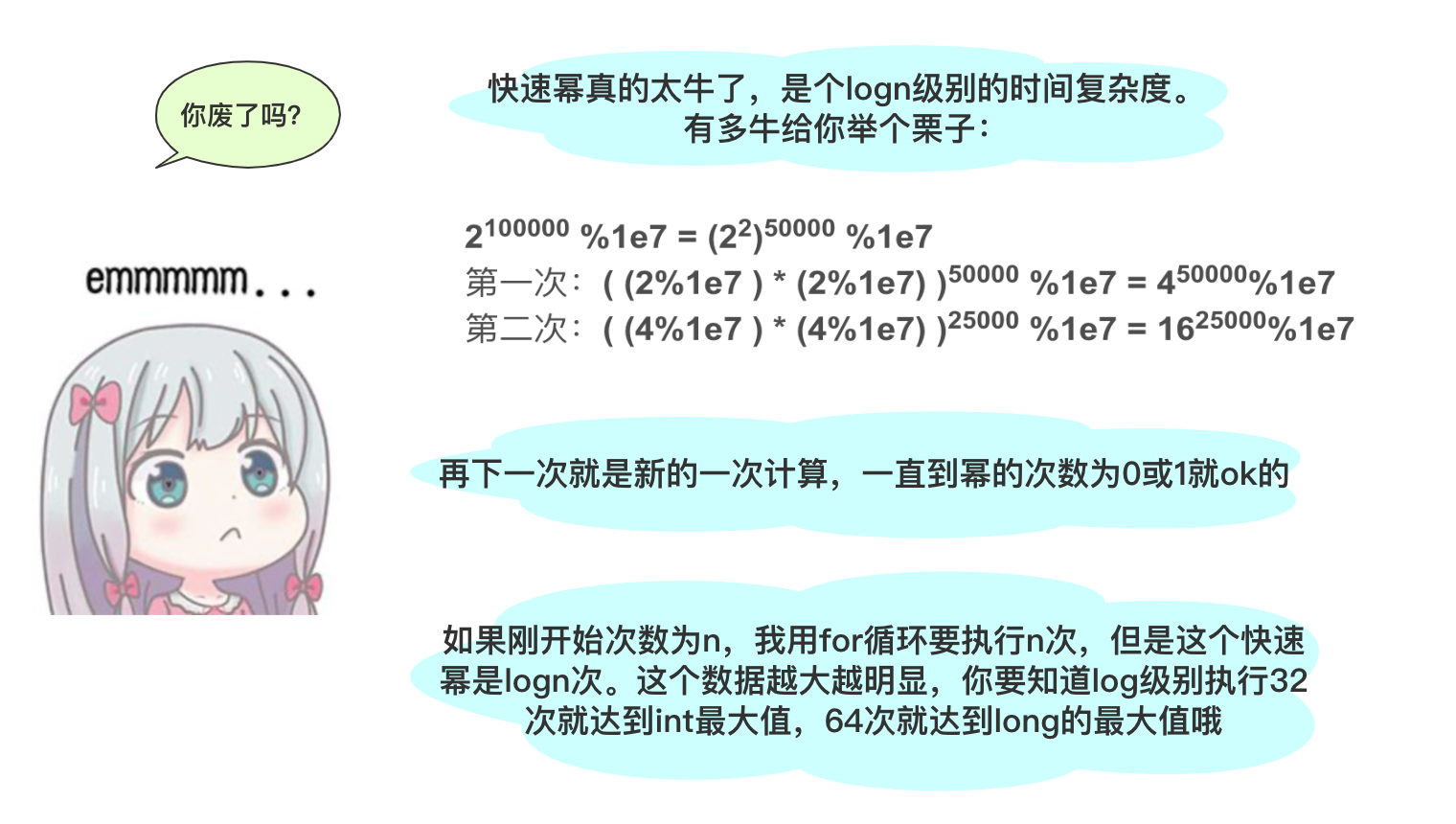## 快速幂实现``````2*2*2*2*2=2 * (2*2*2*2) 奇数问题可以转化为偶数问题。

``````long c=10000007;
public  long divide(long a, long b) {
if (b == 0)
return 1;
else if (b % 2 == 0) //偶数情况
return divide((a % c) * (a % c), b / 2) % c;
else//奇数情况
return a % c * divide((a % c) * (a % c), (b - 1) / 2) % c;
}

``````//求 a^b%1000000007
long c = 1000000007;
public  long divide(long a, long b) {
a %= c;
long res = 1;
for (; b != 0; b /= 2) {
if (b % 2 == 1)
res = (res * a) % c;
a = (a * a) % c;
}
return res;
}

• 为奇数的情况res仅仅是收集相乘那个时候落单的a
• 最终b均会降到1，a最终都会和res相乘，不用担心会漏掉
• 理想状态一直是偶数情况，那最后直接获得a取模的值即可。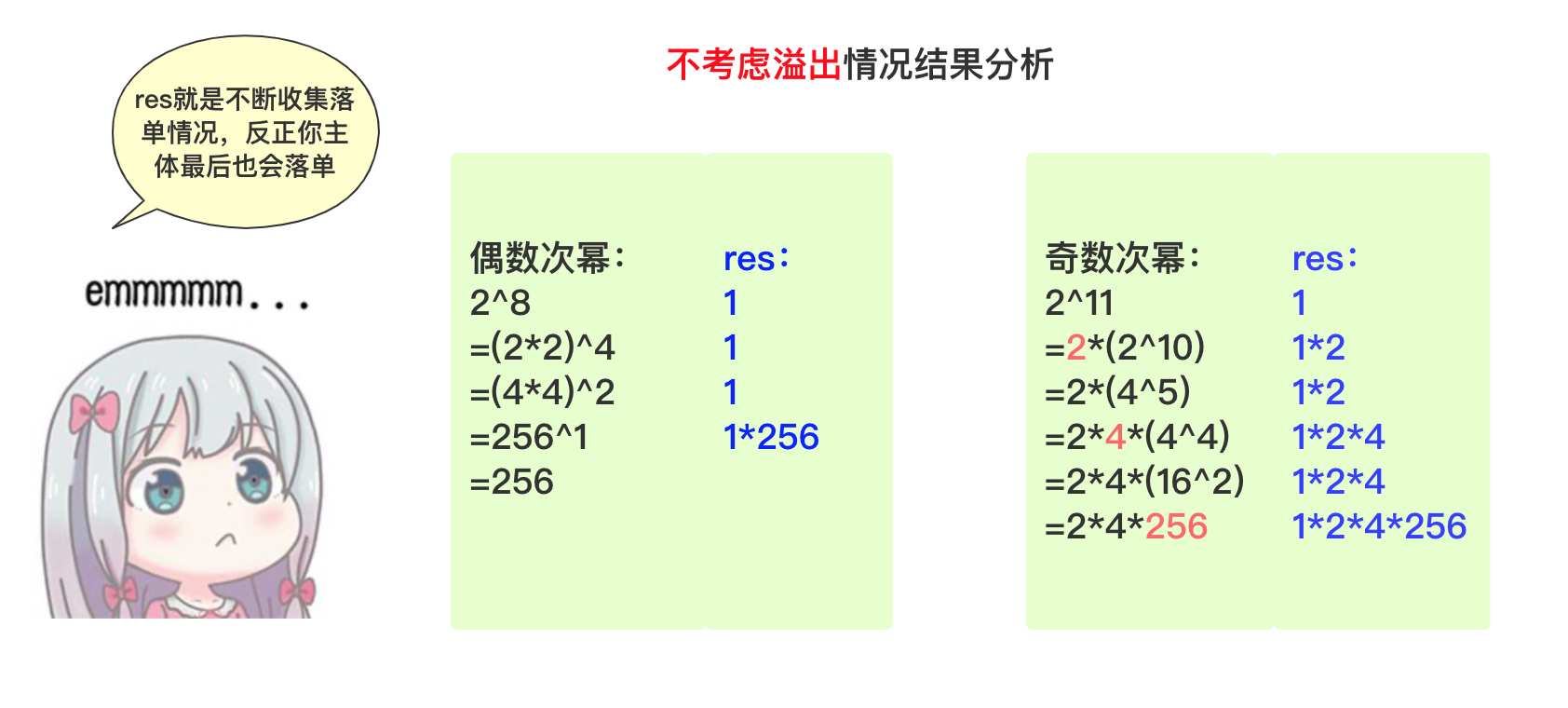## 矩阵快速幂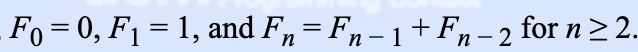``````f(n+1) = f(n)   + f(n-1)
f(n)   = f(n)

``````[f(n+1),f(n)]
=[f(n)+f(n-1),f(n)]

[1  1]
=[f(n),f(n-1)]  *
[1  0]

[1  1] [1   1]
=[f(n-1),f(n-2)]*      *
[1  0] [1   1]

=·······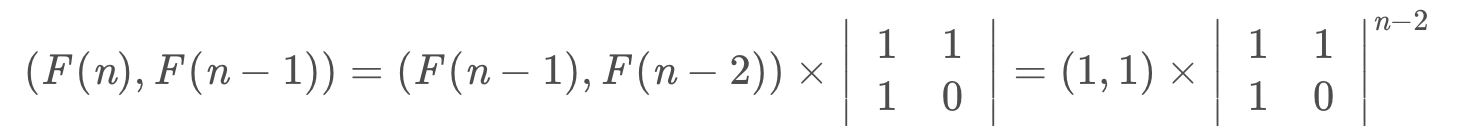``````public int Fibonacci(int n)
{
n--;//矩阵为两项
int a[][]= {{1,1},{1,0}};//进行快速幂的矩阵
int b[][]={{1,0},{0,1}};//存储漏单奇数、结果的矩阵，初始为单位矩阵
int time=0;
while(n>0)
{
if(n%2==1)
{
b=matrixMultiplication(a, b);
}
a=matrixMultiplication(a, a);
n/=2;
}
return b;
}
public  int [][]matrixMultiplication(int a[][],int b[][]){//
int x=a.length;//a.length=b.length 为满足条件
int y=b.length;//确定每一排有几个
int c[][]=new int [x][y];
for(int i=0;i<x;i++)
for(int j=0;j<y;j++)
{
//需要确定每一个元素
//c[i][j];
for(int t=0;t<b.length;t++)
{
c[i][j]+=(a[i][t]%10000)*(b[t][j]%10000);
c[i][j]%=10000;
}
}
return c;
}

## 结语429